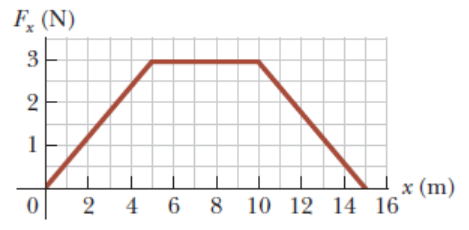# Problem: A particle is subject to a force Fx that varies with position as shown in the following figure. Find the work done by the force on the particle as it moves from x = 10.0 m to x = 15.0 m.

###### FREE Expert Solution

Work done is equal to the area under the curve. The area is triangular.

89% (138 ratings)###### Problem Details

A particle is subject to a force Fx that varies with position as shown in the following figure. Find the work done by the force on the particle as it moves from x = 10.0 m to x = 15.0 m.